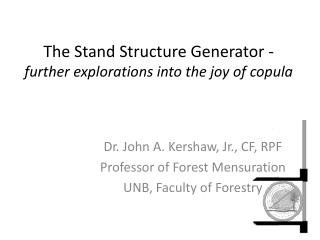DownloadDownload PresentationThe Stand Structure Generator - further explorations into the joy of copula

The Stand Structure Generator - further explorations into the joy of copula

Download PresentationThe Stand Structure Generator - further explorations into the joy of copula

- - - - - - - - - - - - - - - - - - - - - - - - - - - E N D - - - - - - - - - - - - - - - - - - - - - - - - - - -
Presentation Transcript

1. The Stand Structure Generator -further explorations into the joy of copula Dr. John A. Kershaw, Jr., CF, RPF Professor of Forest Mensuration UNB, Faculty of Forestry

2. Genest, C. and MacKay, J. (1987). The Joy of Copulas: The Bivariate Distributions with Uniform Marginals. American Statistician, 40, 280-283.

3. Copula • [kop-yuh-luh] • something that connects or links together

4. Sklar's theorem • Given a joint distribution function H for p variables, and respective marginal distribution functions, there exists a copula C such that the copula binds the margins to give the joint distribution.

5. Gaussian Copula • H(x,y) is a joint distribution • F(x) is the marginal distribution of x • G(y) is the marginal distribution of y • H(x,y) = Cx,y,p[Φ-1(x),Φ-1(y)] • Φ is the cumulative Normal distribution • p is the correlation between x and y • So dependence is specified in the same manner as with a multivariate Normal, but, like all copulae, F() and G() can be any marginal distribution

6. Simulating Spatially Correlated Stand Structures • Start with spatial point process • Mixed Weibull distributions for dbh and height • Correlate dbh, ht, and spatial point process via the Gaussian copula

7. The Stand Structure Generator • Built in R using tcl/tk interface • Spatial Model • Species Model • Correlation Model • Copula Generator • Visualization

8. Structure Generator

9. Spatial Model Lattice Process Thomas Process Easy to add additional point process models

10. Spatial Model • Specify the region, spatial model, and density • Generates the point process • Calculates Voronoi polygons and associated polygon areas • Empirical polygon area distribution is standardized to a mean of 0 and variance of 1 • Std(0,1) is used as Normal marginal for Area

11. Spatial Process

12. Species Distributions

13. Species Distributions • Composition • Mixed Weibull distributions • 2-Parameter, left truncated for DBH • 3-Parameter, reversed for Height

14. Correlations

15. Generation Process • Spatial Pattern -> Voronoi Area -> Std(0,1) area A(0,1) • Randomly sample N(0,1) for DBH D(0,1) • Randomly sample N(0,1) for Height H(0,1) • Correlate [A(0,1) D(0,1) H(0,1)] using the correlation matrix • Choleski’s decomposition • Strip off Normal marginals by applying Inverse Normal: Pr[A(0,1) D(0,1) H(0,1)] • Apply DBH and Height marginal distributions

16. Correlated Pr[A(0,1) D(0,1) H(0,1)]

17. Correlated Spatial Data

18. Visualization • Runs SVS from R to visualize the stand structure

19. Mark Correlations(Observed vs Simulations) Rho_f(r) Distance (r)